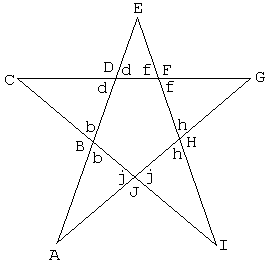# A Problem on a Pentagonal Star

Every one with even the slightest familiarity of the most basic geometric ideas must be able to solve the problem I am going to offer shortly. A proof is therefore supplied only for the completeness' sake. For me, the main attraction of the problem is in that it's formulated for a pentagon, or, more accurately, for a pentagonal star. The greatest part of Planimetry - Plane Geometry - deals with properties of triangle, quadrilateral and sometimes hexagons, like in Pascal's theorem (In a hexagon inscribed in a conic, the three points of intersection of opposite pairs of sides are collinear.) Very few problems deal with other polygons.

Here is then the problem. Let AEICG be a pentagonal star as depicted below.Is it possible that all five inequalities AJ > AB, CB > CD, ED > EF, GF > GH, IH > IJ hold simultaneously?

### Reference

1. D. Fomin, S. Genkin, I. Itenberg, Mathematical Circles (Russian Experience), AMS, 1996### ProofAssume all the inequalities in the formulation of the problem hold. As is well known, in a triangle, a greater angle lies opposite a greater side. Therefore, for angles b, d, f, h, j we have the following chain of inequalities:

b > d > f > h > j > b

The problem and its proof can be adapted to other polygons, but not all. Which?Since the original formulation that included 10 different segments has been reduced to a question about 5 pairs of equal angles, it appears possible to obtain a stronger result. Indeed, let's apply the Law of Sines in each of the five triangles ABJ, BCD, DEF, FGH and HIJ (in all cases the angles are denoted with a single letter with no ambiguity):

 AJ/AB = sin(B)/sin(J) CB/CD = sin(D)/sin(B) ED/EF = sin(F)/sin(D) GF/GH = sin(H)/sin(F) IH/IJ = sin(J)/sin(H)

Multiplying the five identities we get AJ·CB·ED·GF·IH = AB·CD·EF·GH·IJ, which also answers the original question.

The latter identity was first proved by Larry Hoehn (Mathematics Magazine, 66:2 121-123). The above proof is due to Cheng-Shyong Lee (The College Mathematics Journal, 29:2, 1998, 144-145.)